# Basic Tutorial Lesson 6: Exploring Simple Rectifier Circuits Using Ideal Transformers

 Tutorial Project: Exploring Rectifier Circuits Using Ideal Transformers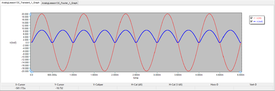Objective: In this project, you will analyze several rectifier circuits in time and Fourier domains. Concepts/Features: Ideal Transformer Tapped Transformer Rectifier Transient Test Fourier Analysis Diode Bridge Minimum Version Required: All versions

## What You Will Learn

In this tutorial you will learn how to use RF.Spice's transformer models. You will build and test half-wave and full-wave rectifier circuits and perform Fourier analysis of the waveforms.

## Building and Testing a Basic Transformer Circuit

The following is a list of parts needed for this part of the tutorial lesson:

Part Name Part Type Part Value
V1 Voltage Source Sinusoidal, Vpeak = 20, f = 1kHz
R1 Resistor 1k
K1 Ideal Transformer n = 2
IN, OUT Voltage Probe Marker N/A

The figure below shows the property dialog of the ideal transformer device. The default value of "Primary-to-Secondary Turns Ratio" is 2, which you will keep for this project.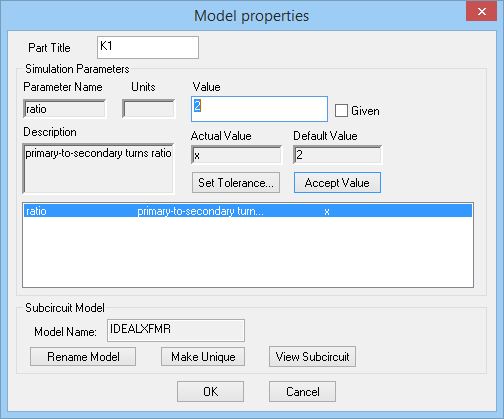The property dialog of the Ideal Transformer.

You can access the "Ideal Transformer" part from the menu item Menu > Parts > Passive Devices > Transformers > Ideal Transformer or simply using the keyboard shortcut K. Place the parts and connect them as shown in the figure below. Make sure to ground the negative pins of the transformer both at the primary and secondary sides.

Run a Transient Test of this circuit with the parameters specified below:

 Start Time 0 5m 10u 1u v(in), v(out)

The results for the input and output voltages are shown in the figure below: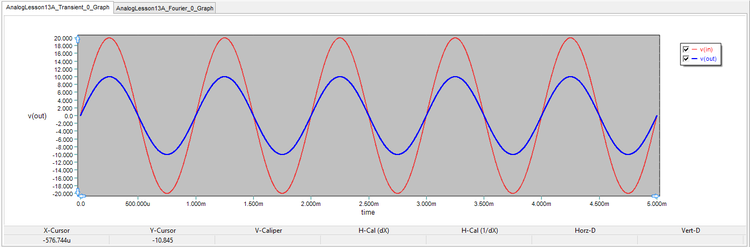The graph of input and output voltages in the transformer circuit.

Next, run another Transient Test of this circuit with different signals to plots:

 Start Time 0 5m 10u 1u i(v1), i(r1)

The results for the input and output currents passing through the voltage source and load resistor, respectively, are shown in the figure below:The graph of input and output currents in the transformer circuit.

It is easy to verify your results with the ideal transformer theory:

$\frac{v_S}{v_P} = \frac{v_{out}}{v_{in}} = \frac{10V}{20V} = \frac{1}{2} = \frac{1}{n}$

$\frac{i_S}{i_P} = \frac{i(R1)}{i(V1)} = \frac{-10mA}{5mA} = -2 = -n$

where vP and vS are the primary and secondary voltages, respectively, and iP and iS are the primary and secondary currents, respectively.

## Running a Fourier Analysis

Your input and output voltages in the circuit of the previous parts are pure single-tone sinusoidal with a frequency of 1kHz. if you perform a Fourier analysis of the output voltage, you would expect to see spectral content only a 1kHz. RF.Spice A/D lets you perform a Fourier analysis in conjunction with its "Transient Test". For this purpose, you need to check the Apply Fourier checkbox in the Transient Test Panel. Then click the Fourier Setup button to open the Fourier Transform Settings dialog. Set the Fundamental Frequency to 1kHz, set the positive output node to "2" for the output voltage and set the reference output node to "0" for the ground.

Run a new Transient Test of this circuit with the parameters specified below:

 Start Time 0 5m 10u 1u v(in), v(out)

At the end of the simulation, an additional bar chart graph is added to the Data Manager window as shown below. Note almost zero spectral contents at DC and all the higher harmonics.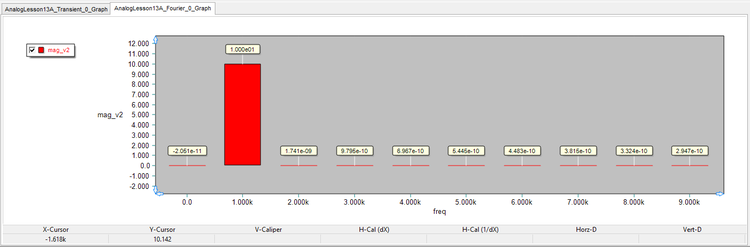The spectral contents of the output voltage in the transformer circuit.

## Building and Testing a Half-Wave Rectifier

The following is a list of parts needed for this part of the tutorial lesson:

Part Name Part Type Part Value
V1 Voltage Source Sinusoidal, Vpeak = 1, f = 1kHz
R1 Resistor 1k
K1 Ideal Transformer n = 2
D1 Generic Diode Defaults
IN, OUT Voltage Probe Marker N/A

By inserting a diode before the load resistor, you can turn the your circuit to a half-wave rectifier as shown in the figure below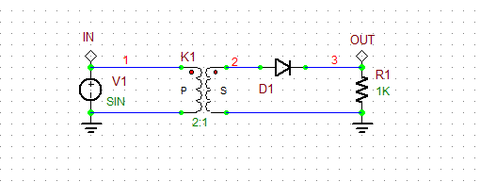The schematic of a half-wave rectifier circuit.

Run a Transient Test of this circuit with the parameters specified below:

 Start Time 0 5m 10u 1u v(in), v(out) Fund. Freq.: 1kHz, Signal: Node 3

The results for the input and output voltages as well as the spectral contents of the output signal are shown in the figures below. The output voltage samples the positive half-cycles of the input sinusoid. As you would expect, the output signal has significant spectral contents at DC and higher harmonics.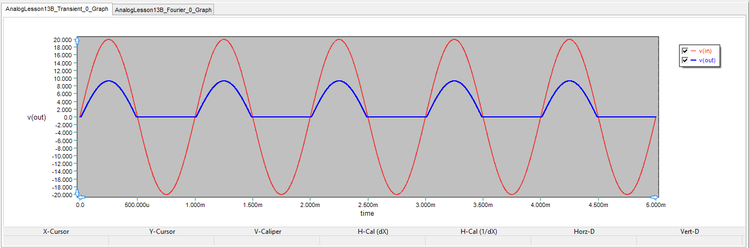The graph of input and output voltages in the half-wave rectifier circuit.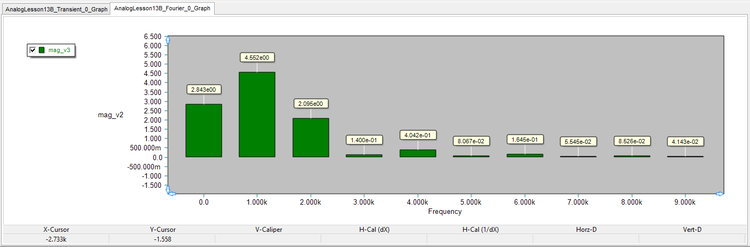The spectral contents of the output voltage in the half-wave rectifier circuit.

## Building and Testing a Full-Wave Bridge Rectifier

The following is a list of parts needed for this part of the tutorial lesson:

Part Name Part Type Part Value
V1 Voltage Source Sinusoidal, Vpeak = 1, f = 1kHz
R1 Resistor 1k
K1 Ideal Transformer n = 2
U Diode Bridge Defaults
IN, OUT Voltage Probe Marker N/A

You can access the diode bridge part form the menu item Menu > Parts > Active Devices > Diodes > Diode Bridge. Place and connect the parts as shown below: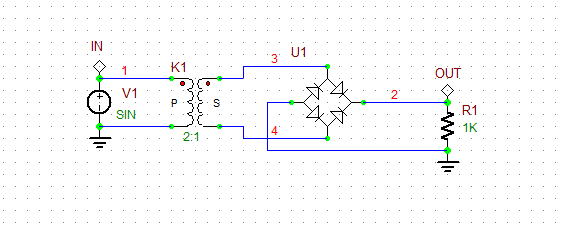The schematic of a full-wave bridge rectifier circuit.

Run a Transient Test of this circuit with the parameters specified below:

 Start Time 0 5m 10u 1u v(in), v(out) Fund. Freq.: 1kHz, Signal: Node 2

The results for the input and output voltages as well as the spectral contents of the output signal are shown in the figures below. It is interesting to note that there is very negligible spectral content at the fundamental frequency of 1kHz. Instead, there is significant spectral content at the second harmonic 2kHz as well as at DC and higher even harmonics. The full-wave rectifier in effect doubles the frequency of the output voltage.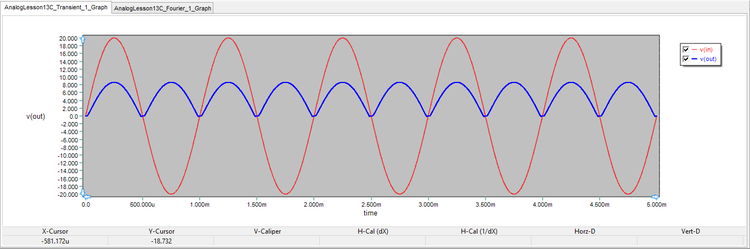The graph of input and output voltages in the full-wave bridge rectifier circuit.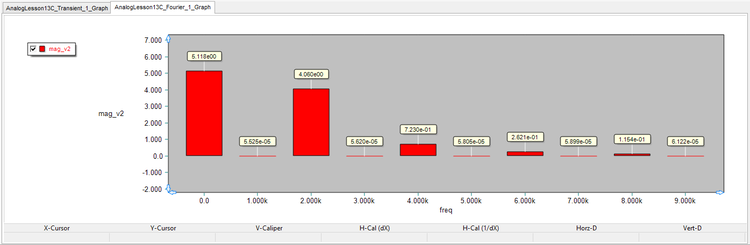The spectral contents of the output voltage in the full-wave bridge rectifier circuit.

## Building and Testing a Full-Wave Rectifier With a Center-Tapped Transformer

The following is a list of parts needed for this part of the tutorial lesson:

Part Name Part Type Part Value
V1 Voltage Source Sinusoidal, Vpeak = 1, f = 1kHz
R1 Resistor 1k
K1 Ideal Center-Tapped Transformer with Push-Pull Output n = 2
D1 - D2 Generic Diode Defaults
IN, OUT Voltage Probe Marker N/A

In this part of the tutorial lesson, you will used a center-tapped transformer. You need an ideal transformer with a single input winding and two output windings. You can get this from the menu item Menu > Parts > Passive Devices > Transformers > Ideal Center-Tapped Transformer with Push-Pull Output. Place and connect the two diodes at the secondary side of the transformer and ground the transformer's center tap as shown in the figure below: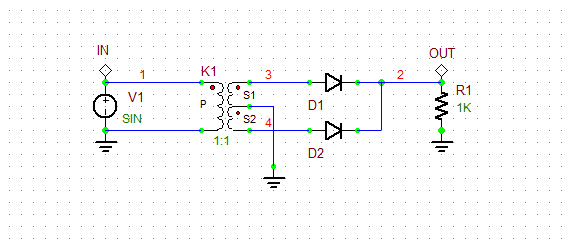The schematic of a full-wave rectifier circuit with a center-tapped transformer.For the ideal center-tapped transformer with push-pull output, the ratio parameter denotes the ratio of the primary winding turns to the secondary half-winding turns. For the ideal center-tapped transformer with push-pull input, the ratio parameter denotes the ratio of the primary half-winding turns to the secondary winding turns.

Run a Transient Test of this circuit with the parameters specified below:

 Start Time 0 5m 10u 1u v(in), v(out) Fund. Freq.: 1kHz, Signal: Node 2

The results for the input and output voltages as well as the spectral contents of the output signal are shown in the figures below. Note how this full-wave rectifier provide better rectification due to the center-tapped transformer in comparison with the bridge rectifier. The spectral contents in this case are very similar to those of the bridge rectifier.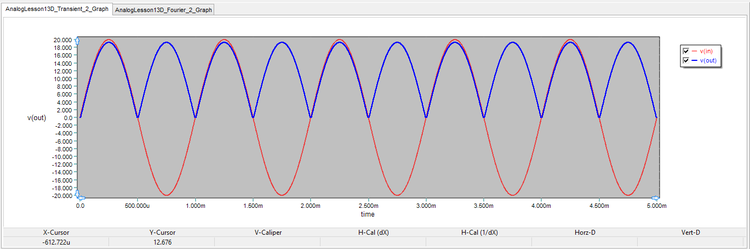The graph of input and output voltages in the full-wave rectifier circuit with a center-tapped transformer.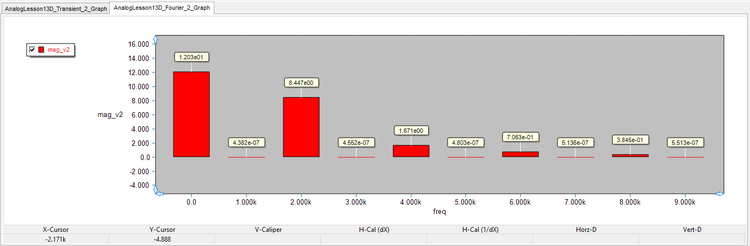The spectral contents of the output voltage in the full-wave rectifier circuit with a center-tapped transformer.